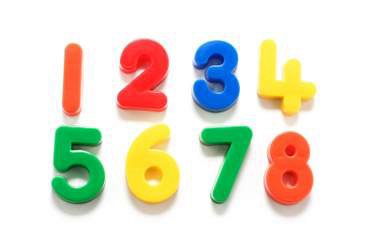• Save

Maths questions series #4

3 years ago 15.8K ViewsVery important Maths questions for SSC CGL-13 Series#3

61. If O is the circumcentre of ?ABC and ?OBC = 350, then the ?BAC is equal to-

a. 550
b. 1100
c. 700
d. 350

62.. If I is the incentre of ? ABC and angle BIC = 1350, then &Delta ABC is-

a. Acute angled
b. Equilateral
c. Right angled
d. Obtuse angled

63. The length of a shadow of a vertical tower is 1//?3 times its height. The angle of elevation of the Sun is-

a. 300
b. 450
c. 600
d. 900

64. The graphs of x +2y =3 and 3x-2y = 1 meet the Y-axis at two points having distance-

a. 8/3 units
b. 4/3 units
c. 1 unit
d. 2 units

65. If x+1/16x = 1, then the value of 64×3 + 1/64×3 is-

a. 4
b. 52
c. 64
d. 76

66. If a, b, c, are three non-zero real numbers such that a + b + c = 0, and b2 ? ca, then the value of a2 + b2 + c2/ b2 –ca is-

a. 3
b. 2
c. 0
d. 1

67. If a4 + a2 b2 + b4 = 8 and a2 + ab + b2 = 4, then the value of ab is-

a. -1
b. 0
c. 2
d. 1

68. If a = 25, b = 15, c = -10, then the value of a3 + b3 + c3 – 3abc/ (a-b)2 + (b-c)2 + (c-a)2 is-

a. 30
b. -15
c. -30
d. 15

69. A, B, C are three points on a circle. The tangent at A meets BC produced at T, angle BTA = 400, angle CAT = 440. The angle subtended by BC at the centre of the circle is-

a. 840
b. 920
c. 960
d. 1040

70. If the length of a chord of a circle at a distance of 12 cm from the Centre is 10 cm, then the diameter of the circle is-

a. 13 cm
b. 15 cm
c. 26 cm
d. 30 cm

71. In &Delta ABC, P and Q are the middle points of the sides AB and AC respectively. R is a point on the segment PQ such that PR : RQ = 1 : 2. If PR = 2 cm, then BC =

a. 4 cm
b. 2 cm
c. 12 cm
d. 6 cm

a. 4 cm
b. 2 cm
c. 12 cm
d. 6 cm

72. The value of cot ?/20 cot 3? /20 cot 5? /20 cot 7? /20 cot 9? /20 is-

a. -1
b. ½
c. 0
d. 1